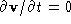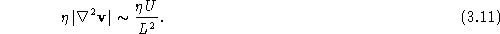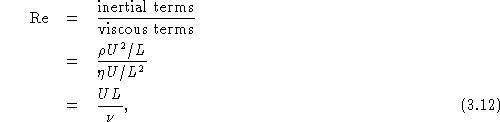Next: Fluid flow at low Up: Real Fluids Previous: The stress tensor and

# The Reynolds number

The Navier-Stokes equation is a rather complicated-looking nonlinear partial differential equation. In order to make some progress in understanding the possible solutions, it is useful to do some dimensional analysis and to make some estimates. For this purpose, we want to compare the inertial terms on the left hand side to the viscous terms on the right hand side. Let's assume that the flow is steady, so that. Let U be a characteristic speed of the flow (usually taken to be the free-stream speed far from the object), and let L be some characteristic dimension of the flow (the typical size of an object in the fluid, say). Then for the inertial term,For the viscous term,Both of these quantities have the same dimension, so we can define a dimensionless number, the Reynolds number  Re, by taking the ratio:with n = h/r the kinematic viscosity.

To get an idea of the magnitude of the Reynolds number, consider a car moving at a speed of 55 mi/hr (= 24 m/s). An appropriate value of L would be the typical width of the car, which I would estimate to be about 1.5 m. The Reynolds number for the flow of air about the car is thenThis is fairly typical--large Reynolds number flow tends to be the rule in most situations.

Vittorio Celli
Sun Sep 28 22:13:11 EDT 1997